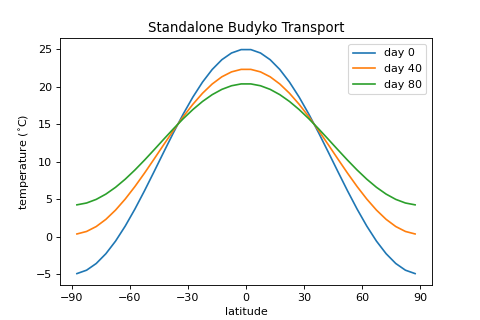# BudykoTransport¶class climlab.dynamics.budyko_transport.BudykoTransport(b=3.81, **kwargs)[source]

calculates the 1 dimensional heat transport as the difference between the local temperature and the global mean temperature.

Parameters

b (float) – budyko transport parameter n - unit: $$\\textrm{W} / \\left( \\textrm{m}^2 \\ ^{\circ} \\textrm{C} \\right)$$ n - default value: 3.81

As BudykoTransport is a Process it needs a state do be defined on. See example for details.

Computation Details: n

In a global Energy Balance Model

$\begin{split}C \\frac{dT}{dt} = R\downarrow - R\uparrow - H\end{split}$

with model state $$T$$, the energy transport term $$H$$ can be described as

$\begin{split}H = b [T - \\bar{T}]\end{split}$

where $$T$$ is a vector of the model temperature and $$\\bar{T}$$ describes the mean value of $$T$$.

For further information see [Budyko_1969].

Example

Budyko Transport as a standalone process:

import climlab
from climlab.dynamics.budyko_transport import BudykoTransport
from climlab import domain
from climlab.domain import field
from climlab.utils.legendre import P2
import numpy as np
import matplotlib.pyplot as plt

# create domain
sfc = domain.zonal_mean_surface(num_lat = 36)

lat = sfc.lat.points

# define initial temperature distribution
T0 = 15.
T2 = -20.
Ts = field.Field(T0 + T2 * P2(np.sin(lat_rad)), domain=sfc)

# create BudykoTransport process
budyko_transp = BudykoTransport(state=Ts)

### Integrate & Plot ###

fig = plt.figure( figsize=(6,4))

for i in np.arange(0,3,1):
ax.plot(lat, budyko_transp.default, label='day %s' % (i*40))
budyko_transp.integrate_days(40.)

ax.set_title('Standalone Budyko Transport')
ax.set_xlabel('latitude')
ax.set_xticks([-90,-60,-30,0,30,60,90])
ax.set_ylabel('temperature ($^{\circ}$C)')
ax.legend(loc='best')
plt.show()Attributes
b

the budyko transport parameter in unit

depth

Depth at grid centers (m)

depth_bounds

Depth at grid interfaces (m)

diagnostics

input

lat

Latitude of grid centers (degrees North)

lat_bounds

Latitude of grid interfaces (degrees North)

lev

Pressure levels at grid centers (hPa or mb)

lev_bounds

Pressure levels at grid interfaces (hPa or mb)

lon

Longitude of grid centers (degrees)

lon_bounds

Longitude of grid interfaces (degrees)

timestep

The amount of time over which step_forward() is integrating in unit seconds.

Methods

 add_diagnostic(self, name[, value]) Create a new diagnostic variable called name for this process and initialize it with the given value. add_input(self, name[, value]) Create a new input variable called name for this process and initialize it with the given value. add_subprocess(self, name, proc) Adds a single subprocess to this process. add_subprocesses(self, procdict) Adds a dictionary of subproceses to this process. compute(self) Computes the tendencies for all state variables given current state and specified input. compute_diagnostics(self[, num_iter]) Compute all tendencies and diagnostics, but don’t update model state. declare_diagnostics(self, diaglist) Add the variable names in inputlist to the list of diagnostics. declare_input(self, inputlist) Add the variable names in inputlist to the list of necessary inputs. integrate_converge(self[, crit, verbose]) Integrates the model until model states are converging. integrate_days(self[, days, verbose]) Integrates the model forward for a specified number of days. integrate_years(self[, years, verbose]) Integrates the model by a given number of years. remove_diagnostic(self, name) Removes a diagnostic from the process.diagnostic dictionary and also delete the associated process attribute. remove_subprocess(self, name[, verbose]) Removes a single subprocess from this process. set_state(self, name, value) Sets the variable name to a new state value. set_timestep(self[, timestep, …]) Calculates the timestep in unit seconds and calls the setter function of timestep() step_forward(self) Updates state variables with computed tendencies. to_xarray(self[, diagnostics]) Convert process variables to xarray.Dataset format.
property b

the budyko transport parameter in unit $$\\frac{\\textrm{W}}{\\textrm{m}^2 \\textrm{K}}$$

Getter

returns the budyko transport parameter

Setter

sets the budyko transport parameter

Type

float